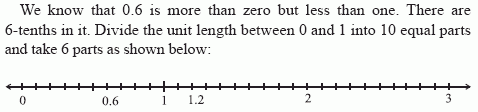Email us to get an instant 20% discount on highly effective K-12 Math & English kwizNET Programs!

Online Quiz (WorksheetABCD)

Questions Per Quiz = 2 4 6 8 10

MEAP Preparation - Grade 5 Mathematics1.72 Decimals on a Number Line

 Read the sequence of decimals given. Find the difference between the second and first decimal. Each succeeding decimal is the difference added to the previous decimal. Example 1: Complete the patters 0.6, 1.2, __Similarly 1.2 is represented. Second decimal - First decimal = 1.2 - 0.6 Difference = 0.6 Hence third decimal = second decimal + difference 1.2 + 0.6 = 1.8 Answer: 1.8 Example 2: Complete the patters 2.3, 2.5, ___, 2.7 Second decimal - First decimal = 2.5 - 2.3 Difference = 0.2 Hence third decimal = second decimal + difference 2.5 + 0.2 = 2.7 Answer: 2.7 Directions: Find the missing decimal in the pattern. Also write at least 10 examples of your own.
 Q 1: Complete the pattern: 8.914, 8.916, 8.918, ____, 8.922.8.9188.9198.9208.921 Q 2: Complete the pattern: 4.1, 4.2, 4.3, ___, 4.54.34.354.54.4 Question 3: This question is available to subscribers only! Question 4: This question is available to subscribers only!